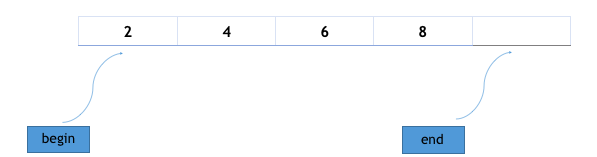×

Search anything:

# begin() and end() in Array C++ STL

#### Software Engineering C++ standard template libraryFREE BOOK -> Problems for the day before your Coding Interview (on Amazon)

In this article, we have explored begin() and end() functions in Array container of C++ STL in depth. We have covered the basic idea of iterators as well as both functions deal with iterators.

Array is a container whose size is fixed. Container is an object that holds same datatype.Array size cannot be allocated dynamically.

SYNTAX of array container:

``````array<object_type, array_size> array_name;
``````

This above code creates an empty array of object_type and its size equals to array_size. However, array can also be created with its elements by using the = operator, here is an example :

``````#include <vector>

int main()
{
array<int, 4> odd_numbers = { 2, 4, 6, 8 };
}
``````

The above code will create an array with its elements i.e.2,4,6,8 .As both functions: begin() and end() return iterators, we will first go through the basic idea behind the different types of iterators and then, explore the two functions in depth.

# Iterators

Iterators are used to point at the memory addresses of STL container.We use iterators to traverse a container like array and to access its elements.They reduce the complexity and execution time of program.

Pointer is one of the form of iterator which point to elements in an array, and can iterate through them using the increment operator (++).

# Types of iterators:

Here, it is the classification of iterators based upon their functionality:

Input Iterators: It is used to read the values from the container.We can retrieve the value from the container by derefrencing an input iterator and it does not change the value of a container.It is also known as one-way iterator as it can be incremented, but cannot be decremented.

Output Iterators: It is an iterator which is used to modify the value in the container.It cannot be used to read the values.We can change the value of the container by dereferencing an output iterator.It is a one-way and write-only iterator as it can be incremented, but cannot be decremented.

Forward Iterator: It is used to read the contents from the beginning to the end of a container.It can also be used to write as it is a multi-pass iterator.It can only move forward because it use only increments operator (++) to move through all the elements of a container.

Bidirectional Iterators: It is used to access the elements in both the directions, i.e., towards the end and towards the beginning.It has all the features of the forward iterator but the difference is that it can also be decremented.

Random-access iterators: It is an iterator that provides random access of an element in any order.It has all the features of a bidirectional iterator but it has one more additional feature, i.e., pointer addition and pointer subtraction that provide random access to an element.

# array::begin()

array::begin() function is a library function of array and it is used to get the first element of the array, it returns an iterator pointing to the first element of the array.

Syntax:

``````array::begin();
``````
• Parameters :No parameters are passed.

• Returns :This function returns a bidirectiona iterator pointing to the first element.

Time complexity

Constant i.e. O(1)

Example

Consider this complete example:

``````// Implementation of begin() function
#include <array>
#include <iostream>
using namespace std;

int main()
{
// declaration of array container
array<int, 5> myarray{ 1, 2, 3, 4, 5 };

// using begin() to print array
for (auto it = myarray.begin(); it! =myarray. end(); ++it)
cout << ' ' << *it;

return 0;
}
``````

Output:

``````1 2 3 4 5
``````

# array::end()

array::end() function is a library function of array and it is used to get the last element of the array, it returns an iterator pointing to the last element of the array.

Syntax:

``````array::end();
``````
• Parameters :No parameters are passed.
• Returns :This function returns a bidirectional iterator pointing to the last element.

Time complexity:

Constant i.e. O(1)

Example

Consider this complete example:

``````// Implementation of end() function
#include <array>
#include <iostream>
using namespace std;

int main()
{
// declaration of array container
array<int, 5> myarray{ 10, 20, 30, 40, 50 };

// using end() to print array
for (auto it = myarray.begin();
it != myarray.end(); ++it)
cout << ' ' << *it;
return 0;
}
``````

Output:

``````10 20 30 40 50
``````

Consider the following code:

``````// Input or array declaration:
array<int,5> arr {10, 20, 30, 40, 50};
// Function call:
auto it=arr.begin();
cout<<*it;
``````

## Question 1

#### What is the output of the above code?

10
20
30
40
Iterator points to the first element of the array i.e. 10.

With this article at OpenGenus, you must have the complete idea of begin() and end() in Array C++ STL. Enjoy.#### Rudrakshi Soni

I am a B.tech student in CSE from JSS Academy of Technical Education, Noida. I love to explore things and a good self-learner. Currently learning new skills and brushing up my programming skills.

begin() and end() in Array C++ STL